Search by Topic

Resources tagged with Investigations similar to National Flags:

Filter by: Content type:
Age range:
Challenge level:

There are 145 results

Broad Topics > Using, Applying and Reasoning about Mathematics > Investigations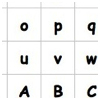It's Times Again

Age 7 to 14 Challenge Level:

Which way of flipping over and/or turning this grid will give you the highest total? You'll need to imagine where the numbers will go in this tricky task!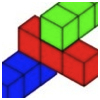Building with Longer Rods

Age 7 to 14 Challenge Level:

A challenging activity focusing on finding all possible ways of stacking rods.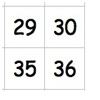So It's Times!

Age 7 to 14 Challenge Level:

How will you decide which way of flipping over and/or turning the grid will give you the highest total?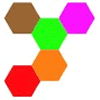Hexpentas

Age 5 to 11 Challenge Level:

How many different ways can you find of fitting five hexagons together? How will you know you have found all the ways?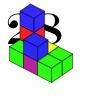28 and It's Upward and Onward

Age 7 to 11 Challenge Level:

Can you find ways of joining cubes together so that 28 faces are visible?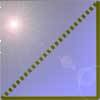Cutting Corners

Age 7 to 11 Challenge Level:

Can you make the most extraordinary, the most amazing, the most unusual patterns/designs from these triangles which are made in a special way?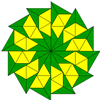Triangle Shapes

Age 5 to 11 Challenge Level:

This practical problem challenges you to create shapes and patterns with two different types of triangle. You could even try overlapping them.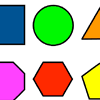Fit These Shapes

Age 5 to 11 Challenge Level:

What is the largest number of circles we can fit into the frame without them overlapping? How do you know? What will happen if you try the other shapes?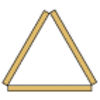Sticky Triangles

Age 7 to 11 Challenge Level:

Can you continue this pattern of triangles and begin to predict how many sticks are used for each new "layer"?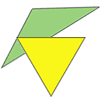Triangle Relations

Age 7 to 11 Challenge Level:

What do these two triangles have in common? How are they related?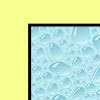All Wrapped Up

Age 7 to 11 Challenge Level:

What is the largest cuboid you can wrap in an A3 sheet of paper?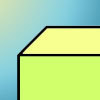Cuboid-in-a-box

Age 7 to 11 Challenge Level:

What is the smallest cuboid that you can put in this box so that you cannot fit another that's the same into it?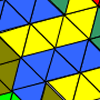Tessellating Transformations

Age 7 to 11 Challenge Level:

Can you find out how the 6-triangle shape is transformed in these tessellations? Will the tessellations go on for ever? Why or why not?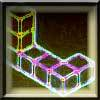Two on Five

Age 5 to 11 Challenge Level:

Take 5 cubes of one colour and 2 of another colour. How many different ways can you join them if the 5 must touch the table and the 2 must not touch the table?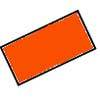Two by One

Age 7 to 11 Challenge Level:

An activity making various patterns with 2 x 1 rectangular tiles.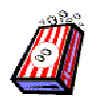Age 7 to 11 Challenge Level:

We went to the cinema and decided to buy some bags of popcorn so we asked about the prices. Investigate how much popcorn each bag holds so find out which we might have bought.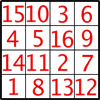Magic Constants

Age 7 to 11 Challenge Level:

In a Magic Square all the rows, columns and diagonals add to the 'Magic Constant'. How would you change the magic constant of this square?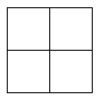Two Squared

Age 7 to 11 Challenge Level:

What happens to the area of a square if you double the length of the sides? Try the same thing with rectangles, diamonds and other shapes. How do the four smaller ones fit into the larger one?Calcunos

Age 7 to 11 Challenge Level:

If we had 16 light bars which digital numbers could we make? How will you know you've found them all?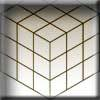Christmas Presents

Age 7 to 11 Challenge Level:

We need to wrap up this cube-shaped present, remembering that we can have no overlaps. What shapes can you find to use?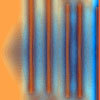Move a Match

Age 7 to 11 Challenge Level:

How can you arrange these 10 matches in four piles so that when you move one match from three of the piles into the fourth, you end up with the same arrangement?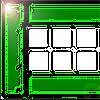Fencing

Age 7 to 11 Challenge Level:

Arrange your fences to make the largest rectangular space you can. Try with four fences, then five, then six etc.2010: A Year of Investigations

Age 5 to 14

This article for teachers suggests ideas for activities built around 10 and 2010.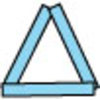Sticks and Triangles

Age 7 to 11 Challenge Level:

Using different numbers of sticks, how many different triangles are you able to make? Can you make any rules about the numbers of sticks that make the most triangles?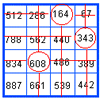Marvellous Matrix

Age 7 to 11 Challenge Level:

Follow the directions for circling numbers in the matrix. Add all the circled numbers together. Note your answer. Try again with a different starting number. What do you notice?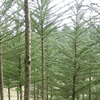It's a Fence!

Age 5 to 11 Challenge Level:

In this challenge, you will work in a group to investigate circular fences enclosing trees that are planted in square or triangular arrangements.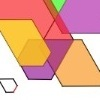The Numbers Give the Design

Age 7 to 11 Challenge Level:

Make new patterns from simple turning instructions. You can have a go using pencil and paper or with a floor robot.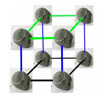More Pebbles

Age 7 to 11 Challenge Level:

Have a go at this 3D extension to the Pebbles problem.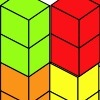Triple Cubes

Age 5 to 11 Challenge Level:

This challenge involves eight three-cube models made from interlocking cubes. Investigate different ways of putting the models together then compare your constructions.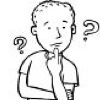Count the Digits

Age 5 to 11 Challenge Level:

In this investigation we are going to count the number of 1s, 2s, 3s etc in numbers. Can you predict what will happen?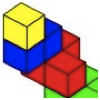Four Layers

Age 5 to 11 Challenge Level:

Can you create more models that follow these rules?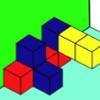Three Sets of Cubes, Two Surfaces

Age 7 to 11 Challenge Level:

How many models can you find which obey these rules?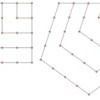Polygonals

Age 7 to 11 Challenge Level:

Polygonal numbers are those that are arranged in shapes as they enlarge. Explore the polygonal numbers drawn here.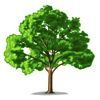How Tall?

Age 5 to 11 Challenge Level:

A group of children are discussing the height of a tall tree. How would you go about finding out its height?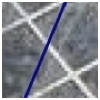Crossing the Town Square

Age 7 to 11 Challenge Level:

This tricky challenge asks you to find ways of going across rectangles, going through exactly ten squares.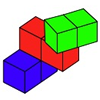Building with Rods

Age 7 to 11 Challenge Level:

In how many ways can you stack these rods, following the rules?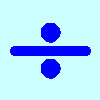Division Rules

Age 7 to 11 Challenge Level:

This challenge encourages you to explore dividing a three-digit number by a single-digit number.Tri.'s

Age 7 to 11 Challenge Level:

How many triangles can you make on the 3 by 3 pegboard?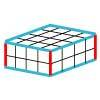Making Cuboids

Age 7 to 11 Challenge Level:

Let's say you can only use two different lengths - 2 units and 4 units. Using just these 2 lengths as the edges how many different cuboids can you make?Egyptian Rope

Age 7 to 11 Challenge Level:

The ancient Egyptians were said to make right-angled triangles using a rope with twelve equal sections divided by knots. What other triangles could you make if you had a rope like this?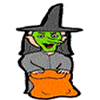Halloween Investigation

Age 7 to 11 Challenge Level:

Ana and Ross looked in a trunk in the attic. They found old cloaks and gowns, hats and masks. How many possible costumes could they make?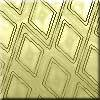Street Party

Age 7 to 11 Challenge Level:

The challenge here is to find as many routes as you can for a fence to go so that this town is divided up into two halves, each with 8 blocks.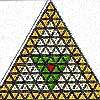The Great Tiling Count

Age 7 to 11 Challenge Level:

Compare the numbers of particular tiles in one or all of these three designs, inspired by the floor tiles of a church in Cambridge.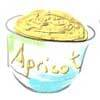Ice Cream

Age 7 to 11 Challenge Level:

You cannot choose a selection of ice cream flavours that includes totally what someone has already chosen. Have a go and find all the different ways in which seven children can have ice cream.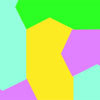Escher Tessellations

Age 7 to 11 Challenge Level:

This practical investigation invites you to make tessellating shapes in a similar way to the artist Escher.Cubes

Age 7 to 11 Challenge Level:

How many faces can you see when you arrange these three cubes in different ways?Little Boxes

Age 7 to 11 Challenge Level:

How many different cuboids can you make when you use four CDs or DVDs? How about using five, then six?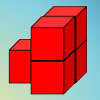Age 7 to 11 Challenge Level:

How can you arrange the 5 cubes so that you need the smallest number of Brush Loads of paint to cover them? Try with other numbers of cubes as well.3 Rings

Age 7 to 11 Challenge Level:

If you have three circular objects, you could arrange them so that they are separate, touching, overlapping or inside each other. Can you investigate all the different possibilities?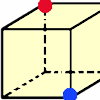Redblue

Age 7 to 11 Challenge Level:

Investigate the number of paths you can take from one vertex to another in these 3D shapes. Is it possible to take an odd number and an even number of paths to the same vertex?